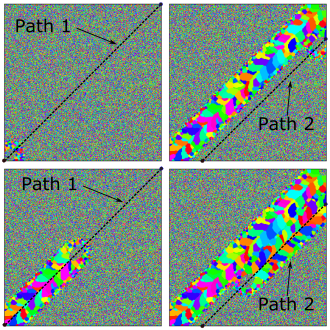SPPARKS Website - SPPARKS Documentation - SPPARKS Commands

### am path_layer command

Syntax:

```am path_layer layer_id num_paths N path_ids (tuple paths_ids) thickness t
```
• layer_id = integer identification number for this path_layer
• num_paths = required keyword
• N = integer number of paths specified on this layer
• path_ids = required keyword
• (tuple path_ids) = tuple array of path_ids corresponding am path specifications elsewhere in script
• thickness = required keyword
• t = thickness in sites of layer

Examples

```variable X0 equal         0.0
variable Y0 equal         0.0
variable X1 equal        500.0
variable Y1 equal        500.0
variable X2 equal        106.1
variable Y2 equal         0.0
variable X3 equal        500.0
variable Y3 equal        393.9
am path 1 start \$X0 \$Y0 end \$X1 \$Y1 speed 9
am path 2 start \$X3 \$Y3 end \$X2 \$Y2 speed 9
am path_layer 1 num_paths 2 path_ids 1 2 thickness 1
```

Description:

This command is used by am/ellipsoid, and potts/am/weld applications to specify raster patterns on path build layers. Multiple path_layers can be defined in a single input file, using unique id values. Although simple build simulations can be conducted using this command by manually writing scripts that use am path_layer, it is expected that this command will generally be automatically created for the purpose of handling more complex geometries.

The num_paths and path_ids keywords are used to create the layer with thickness t. The build layer thickness is specified in units of sites.

In example above, two unique am paths are created; these paths are referenced in the am path_layer command. This example is also illustrated for am_path,Restrictions:

This command can only be used as part of apps am/ellipsoid or potts/am/weld applications.

Related commands:

Default: none